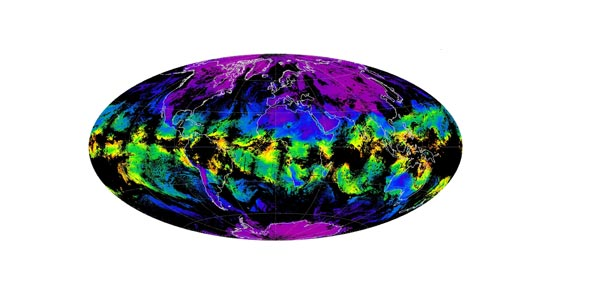# Chpt. 4 - Section A: Gases In The Atmosphere

16 Questions | Total Attempts: 129SettingsChapter 4, Section A: Gases in the Atmosphere

• 1.
This gas is the most abundant in the atmosphere.
• A.

Nitrogen

• B.

Hydrogen

• C.

Oxygen

• D.

Argon

• 2.
Air is best described as...
• A.

Pure substance

• B.

Compound

• C.

Element

• D.

Solution

• 3.
You have 1.42 L of a gas exerting a pressure of 125 kPA. Assuming constant temperature what pressure will the gas exert if its volume is reduced to 0.853L? (Boyle's Law: P1V1 = P2V2)
• A.

75.1 kPA

• B.

208 kPA

• C.

151 kPA

• D.

• 4.
Which of the following terms best describes the relationship between pressure and volume? (Boyle's Law)
• A.

Inversely related

• B.

Directly related

• C.

Complimentary

• D.

Indirectly related

• 5.
Which of the following terms best describes the relationship between temperature and volume?
• A.

Inversely related

• B.

Directly related

• C.

Complimentary

• D.

Indirectly related

• 6.
A temperature of 373 K is equal to what temperature on the Celsius scale?
• A.

0 degrees Celsius

• B.

100 degrees Celsius

• C.

373 degrees Celsius

• D.

646 degrees Celsius

• 7.
What causes pressure inside a tire?
• A.

Gas molecules colliding with each other

• B.

Gas molecules colliding with the wall of the tire

• C.

The diffusion of the gases

• D.

Constant changing of temperature

• 8.
A sample of oxygen occupies 400mL when the pressure is 800mm Hg. If the temperature is held constant, what is the volume that the gas will occupy if the pressure decreases to 400mm Hg? (Boyle's Law: P1V1 = P2V2)
• A.

200mL

• B.

800mL

• C.

1600mL

• D.

2400mL

• 9.
To pop popcorn, you need to increase the temperature, which causes an increase in
• A.

The pressure of the water vapor inside the popcorn kernels

• B.

The volume of the liquid water inside the popcorn kernels

• C.

The mass of the water vapor inside the popcorn kernels

• D.

The solubility of the water vapor inside the popcorn kernels

• 10.
A gas is collected at 600. mm Hg and 300. K. What would the pressure be at 350 K (at constant volume)? (Gay Lussac's Law: P1/T1 = P2/T2)
• A.

175 mm Hg

• B.

300. mm Hg

• C.

514 mm Hg

• D.

700. mm Hg

• 11.
A gas has a pressure of 801 mm Hg at 349 K. If the pressure is reduced to 701 mm Hg, what is the new temperature? (Gay Lussac's Law: P1/T1 = P2/T2)
• A.

250 K

• B.

305 K

• C.

399 K

• D.

802 K

• 12.
Calculate the pressure in kilopascals exerted by a textbook on the desk. The dimensions of the textbook are 24.0 cm by 18.0 cm and the force it exerts is 3.00 Newtons. (Pressure = Force/Area)
• A.

69.4 kPa

• B.

0.0694 kPa

• C.

0.0144 kPa

• D.

14.4 kPa

• 13.
If temperature is held constant, decreasing the volume of a sample of gas will cause its pressure to..
• A.

Decrease

• B.

Increase

• C.

Remain the same

• 14.
In the relationship described by Boyle's law, which property must remain constant?
• A.

Pressure

• B.

Temperature

• C.

Volume

• 15.
When air is removed from a bell jar with a vacuum pump reducing the pressure in the bell jar (at constant temperature), a sealed inflated balloon inside the bell jar
• A.

Gets smaller

• B.

Gets larger

• C.

Stays the same size

• 16.
In the relationship described by Charles' Law, which property must remain constant?
• A.

Pressure

• B.

Temperature

• C.

Volume

Related TopicsBack to top